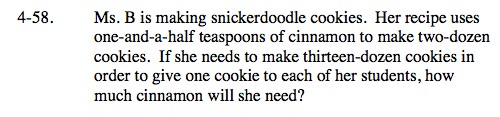### Home > CAAC > Chapter 4 > Lesson 4.1.6 > Problem4-58

4-58.Use proportions to solve this problem. Assign a variable to the unknown number of teaspoons of cinnamon.
For this case, we will use the variable n.

Multiply both sides by 2.

Multiply both sides by 13.

Divide by 2 on both sides.

Remember to use the conversion factor to determine how many tablespoons and teaspoons Ms. B will need.

$\frac{ 1 \frac{ 1 }{2 } \text{ teaspoons}}{ 2\text{ dozen cookies}}= \frac{ n\text{ teaspoons}}{ 13\text{ dozen cookies}}$

$\frac{ 1 \frac{ 1 }{2 } }{2 }= \frac{ n }{13 }$

$1 \frac{ 1 }{2 } = \frac{ 2n }{13 }$

19.5 = 2n

$n=9.75 \text{ or }9 \frac{3}{4} \text{ teaspoons}$